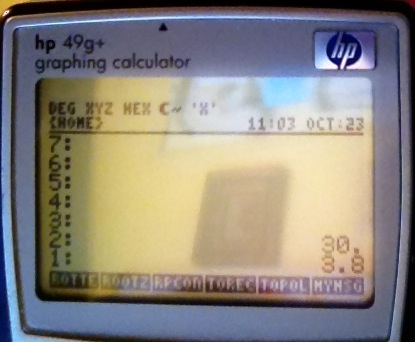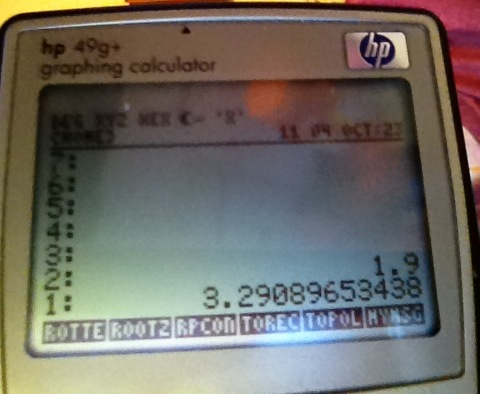## Monday, October 24, 2011

### RPL Programming Tutorial - Part 16 - HP 49g+/50g: Choose Box

Giving Yourself Choices

Welcome to Part 16 of the RPL programming tutorial. Today we will work with CHOOSE boxes.

Note: The CHOOSE box is not available on the HP 48S and HP 28C.

The first two programs, TOREC and TOPOL, will be the subroutines that will be used in a third program, RPCONV.

TOREC: converts polar coordinates to rectangular coordinates

Level 2: θ to y
Level 1: r to x

y = r sin( θ )
x = r cos( θ )

TOPOL: converts rectangular coordinates to polar coordinates

Level 2: y to θ
Level 1: x to r

r = abs(x + yi) = √(x^2 + y^2)
θ = arg(x + yi) = arctan(y/x) where 0 ≤ θ ≤ π (radians) or 0 ≤ θ ≤ 180º (degrees)

The CHOOSE Command

Keystroke sequence for CHOOSE: [LS] [EVAL] (PRG) [NXT] [F5] (IN) [F3] (CHOOS)

Format:

"title of the choose box"
{ {"selection 1" program 1}
{"selection 2" program 2}
...
{"selection n" program n} }
1 CHOOSE

If a selection is made, 1 is returned. If the selection is canceled, 0 is returned. This requires further processing. One way to handle the output of the choose box is:

"title of the choose box"
{ {"selection 1" program 1}
{"selection 2" program 2}
...
{"selection n" program n} }
1 CHOOSE
IF 1 ==
THEN EVAL
ELSE KILL
END

The KILL command stops program execution.

Keystroke sequence for KILL: [LS] [EVAL] (PRG) [NXT] [NXT] [F3] (RUN) [F6] (EVAL)

The Program TOREC

Keystrokes:

[RS] [ + ] ( << >> )
[LS] [EVAL] (PRG) [F1] (STACK) [NXT] [NXT] [F1] (DUP2)

* DUP2 duplicates the contents on Levels 1 and 2
[NXT] [NXT] [F2] (SWAP)
* go the SWAP command from DUP2
[SIN] [ x ] [RS] [ENTER] (→NUM)
* Calculate y = r sin( θ )
[F6] (UNROT)
* rolls down a 3-level stack
[F2] (SWAP) [COS] [ x ] [RS] [ENTER] (→NUM) [ENTER]
* Calculate x = r sin( θ )

[ ' ] [ALPHA] [ALPHA] [COS] (T) [ ' ] (O) [ √ ] (R) [F5] (E) [F3] (C) [ENTER] [STO>]

The completed program:

<< DUP2 SWAP SIN * →NUM UNROT SWAP COS * →NUM >>

The Program TOPOL

Keystrokes:

[RS] [ + ] ( << >> )
[LS] [EVAL] (PRG) [F1] (STACK) [F2] (SWAP)
[LS] [TOOL] ( i ) [ x ] [ + ]

* Forms the complex number x + yi
[F1] (DUP) [RS] [ ÷ ] (ARG) [RS] [ENTER] (→NUM)
* Calculate θ = arg (x + yi)
[F2] (SWAP) [LS] [ ÷ ] (ABS) [RS] [ENTER] (→NUM) [ENTER]
* Calculate r = abs (x + yi)

[ ' ] [ALPHA] [ALPHA] [COS] (T) [ ' ] (O) [SYMB] (P) [ ' ] (O) [NXT] (L) [ENTER] [STO>]

The completed program:

<< SWAP i * + DUP ARG →NUM SWAP ABS →NUM >>

Now that TOREC and TOPOL are complete, let's do the main program, RPCONV. We made TOREC and TOPOL global programs, so they can be used on their own.

The Program RPCONV

[RS] [ + ] ( << >> )
[RS] [ x ] ( " " )
[ALPHA] [ALPHA] [F3] (C) [LS] [ALPHA] [ ' ] (o) [EVAL] (n) [EEX] (v) [F5] (e) [ √ ] (r) [COS] (t) [F5] (e) [ √ ] (r) [LS] [ALPHA] [ALPHA]

* Titles the choose box "Converter"
[SPC] [LS] [ + ] ( { } )
[LS] [ + ] ( { } )
[RS] [ x ] ( " " ) [ALPHA] [ALPHA] [RS] [ 0 ] ( →) [SYMB] (P) [ ' ] (O) [NXT] (L) [F1] (A) [ √ ] (R) [ALPHA]
[ → ] [RS] [ + ] ( << >> ) [ALPHA] [ALPHA] [COS] (T) [ ' ] (O) [SYMB] (P) [ ' ] (O) [NXT] (L) [ALPHA]

* First menu choice, →POLAR. TOPOL is typed.
[ → ] [ → ] [ →] [ → ]
[LS] [ + ] ( { } )
[RS] [ x ] ( " " ) [ALPHA] [ALPHA] [RS] [ 0 ] ( → ) [ √ ] (R) [F5] (E) [F3] (C) [COS] (T) [ALPHA]
[ → ] [RS] [ + ] ( << >> ) [ALPHA] [ALPHA] [COS] (T) [ ' ] (O) [ √ ] (R) [F5] (E) [F3] (C) [ALPHA]

* Second menu choice, →RECT. TOREC is typed.
[ → ] [ → ] [ → ] [ → ] [ → ]
1 [LS] [EVAL] (PRG) [NXT] [F4] (IN) [F3] (CHOOS)

* Exits the nested list and the CHOOSE command
[LS] [EVAL] (PRG) [F3] (BRCH) [RS] [F1] (IF)
* Enters the IF-THEN-ELSE-END structure
1 [LS] [EVAL] (PRG) [F3] (TEST) [F1] ( == )
[ ↓ ] [EVAL]
[ ↓ ] [LS] [EVAL] (PRG) [NXT] [NXT] [F3] (RUN) [F6] (KILL)
[ENTER]

* finishes the CHOOSE structure and terminates program entry

[ ' ] [ALPHA] [ALPHA] [ √ ] (R) [SYMB] (P) [F3] (C) [ ' ] (O) [EVAL] (N) [EEX] (V) [ENTER] [STO>]

The completed instructions:

<< "Converter"
{ { "→POLAR" << TOPOL >> }
{ "→RECT" << TOREC >> } }
1 CHOOSE
IF 1 ==
THEN EVAL
ELSE KILL
END >>

Instructions:

Rectangular to Polar Conversion

1. Enter y
2. Press [ENTER]
3. Enter x
4. Run RPCONV
5. Select the →POLAR option

Polar to Rectangular Conversion

1. Enter θ
2. Press [ENTER]
3. Enter r
4. Run RPCONV
5. Select the →RECT option

Examples (the calculator is in Degrees mode):

1. Convert r = 3.8, θ = 30º to rectangular coordinates.

Result: x ≈ 3.2909, y ≈ 1.9000

2. Convert x = 3.2, y = 6.3 to polar coordinates.

Result: r ≈ 7.0661, θ ≈ 63.0723º

Below are screen shots of Example 1.Next time we will explore system flags.

Have a great day,

Eddie

This tutorial is property of Edward Shore. Mass reproduction and distribution requires express permission of the author.

### Swiss Micros DM16L: Advanced Boolean and Factorial (up to 20)

Swiss Micros DM16L:   Advanced Boolean and Factorial (up to 20) Introduction The program listing, for the Swiss Micros DM16L and Hewlett Pac...